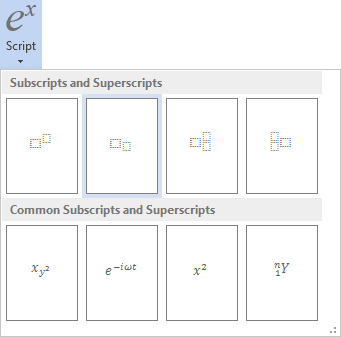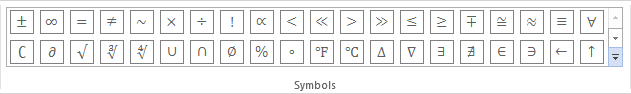# How to insert an equation with matrix

Word
This tip display how to insert an equation for example, the Electromagnetic tensor.

Do the following (how to add an equation in your document, see Working with Microsoft Equation):

In the Professional presentation:

2.   On the Equation Tools Design tab, in the Structures group, click the Script button:In the Script list choose Subscript:3.   In the base box of script enter F and in the lower right box of script:

• On the Equation Tools Design tab, in the Symbols group, click the More button:• In the list of symbols chooseand then.

4.   Enter =.

5.   On the Equation Tools Design tab, in the Structures group, click the Bracket button. In the Bracket list choose round brackets:6.   On the Equation Tools Design tab, in the Structures group, click the Matrix button. In the Matrix list choose 2x2 Empty Matrix or 3x3 Empty Matrix:7.   To increase a count of columns or/and rows of your matrix, you can right-click in it and in the popup menu in the Insert list, choose what and how you want to increase:8.   Enter in some positions zero, for other positions use Subscript and Fraction:In the Linear presentation:

1.   In your own equation enter F_.

2.   On the Equation Tools Design tab, in the Symbols group, choose(or \alpha) and then(or \beta).

3.   Enter =. Then you enter an equal symbol, this linear formula transformed to the professional format:4.   In the brackets, enter the following:

(\matrix(0&E_x/c&E_y/c&E_z/c@(-E_x)/c&0&-B_z&B_y@(-E_y)/c&B_z&0&-B_x@(-E_z)/c&-B_y&B_x&0));

where \matrix is a command to create a matrix, & divides elements to columns in one row, @ divides rows in the matrix. Every time then you enter special symbol, this linear formula transformed to the professional format.

Note: You can change spaces and alignment of your matrix, for more details see Adjusting spacing and alignment in an equation.